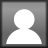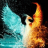November 20

# Tonight’s Math Homework

Here is the online homework 4A and 4B  voted for tonight. This task is just like the other tasks we have done in class. Try and find as many different ways to combine these numbers using addition, subtraction, multiplication, and division to get 24 as an answer.  You may only use each number once and you cannot combine the numbers to make a two-digit number. You can write your questions down on paper or you can show your answers in the comment section of this post. Have fun!Tags:

Posted November 20, 2019 by jbennett31 in category General Studies, Homework

## 6 thoughts on “Tonight’s Math Homework”

1.Oleg Tupitsyn

Thank you, Julie! Indeed a fun way to learn Math. While Joel is struggling through the task, I have found some ambiguities. Please enlighten me with the following:

1. Are those numbers values or digits (may they enter combinations with themselves or should they be used as-are for math operations)?

2. What is the meaning of “multiplication, and multiplication” – was the second one meant to read “division”?

3. Assuming that 7, 5, 8, 4 are values, – are they allowed to be used more than once in a calculation?

4. May one use more than one math operation (+, -, X, 🙂 in a single calculation?

5. Are parenthesis allowed to define the order of precedence?

Thank you!

Oleg

1.jbennett31 (Post author)

Hi Oleg,
The numbers are the values and they can only be used once. The numbers cannot be combined to make a two-digit number. One can use more than one operation in a calculation. Parentheses may also be used to define the order of precedence. Please keep in mind Joel has not used parentheses in class yet. I can teach him how to use them if you want.😊
Happy calculating!
Julie

2.Adam Tsehmaister

5 + 4 + 8 + 7 = 24
(5+7) x (8:4) = 12 x 2 = 24
(7-5) x (8+4) = 2×12 = 24

3.Lily

8+4+7+5=24
(7+5)x(8/4)=12×2=24
4!x(8-7)^5=(4x3x2x1)x(1x1x1x1x1)=24×1=24

4.Benny

5+8+7+4=24
(8/4)*(5+7)=2*12=24
[8-(7-5)]*4=[8-2]*4=6*4=24
(7-4)*8=3*8=24

5.Adam Levitan

#1: 7 x 7 – 4 – 8 – 7 – 5 + 4 – 4 = 24

7 x 7 = 49 – 4 = 45 – 8 = 37 – 8 = 29 – 5 = 24

#2: 7 + 8 + 5 + 4 = 24

7 + 8 = 15 + 5 = 20 + 4 = 24